# Down the rabbit hole with R functions

I had a friend the other day ask me about modifying the plot that goes with R’s boxCox function. In particular they had multiple plots, and wanted to make the Y axes consistent between the different dependent variables. So for a typical R base plot call, you can specify `ylim = c(whatever_low, whatever_high)`, but if you look at function in the end it does not let you do this yourself (it fixes ylim based on the log-likelihood range.

``````library(car)
data(trees)
# Making a second Y variable for illustration later
trees\$V2 <- trees\$Volume*2 + 3*rnorm(nrow(trees))

# Original function, https://rdrr.io/rforge/car/man/boxCox.html
orig_output <- with(trees, boxCox(Volume ~ log(Height) + log(Girth), data = trees))``````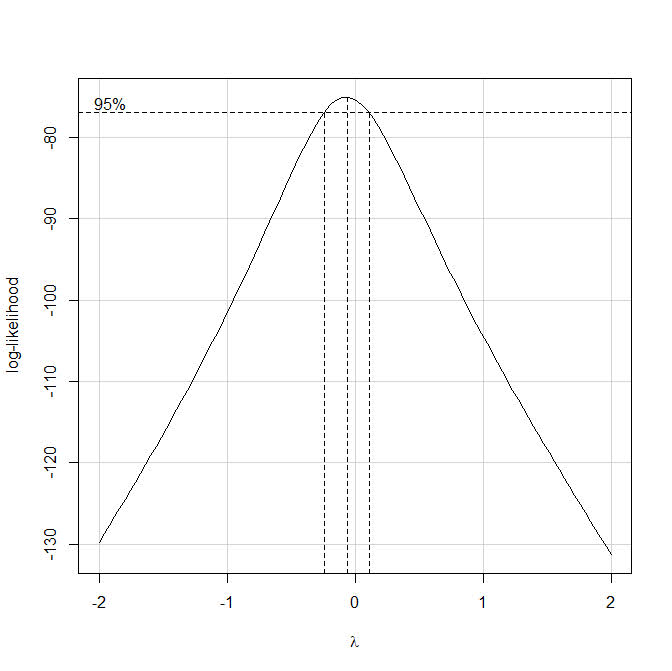So if we look at the `orig_output` object, it gives us the x and y values for the above plot, but it does not give us the dashed line locations in the plot.Typically here I would type out `boxCox` without the parenthesis at the prompt to get the function definition. That does not quite work here, as it is unhelpful and just gets us the message `useMethod(boxCox)`. From here we can do the function `method(boxCox)` to help slightly more – we can see that the `boxCox` function really has 3 different functions, that depend on the original input.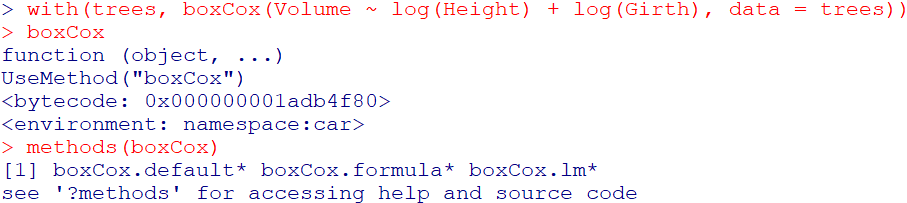Here we are specifying the formula interface to the function call, so lets look at `getAnywhere(boxCox.formula)`: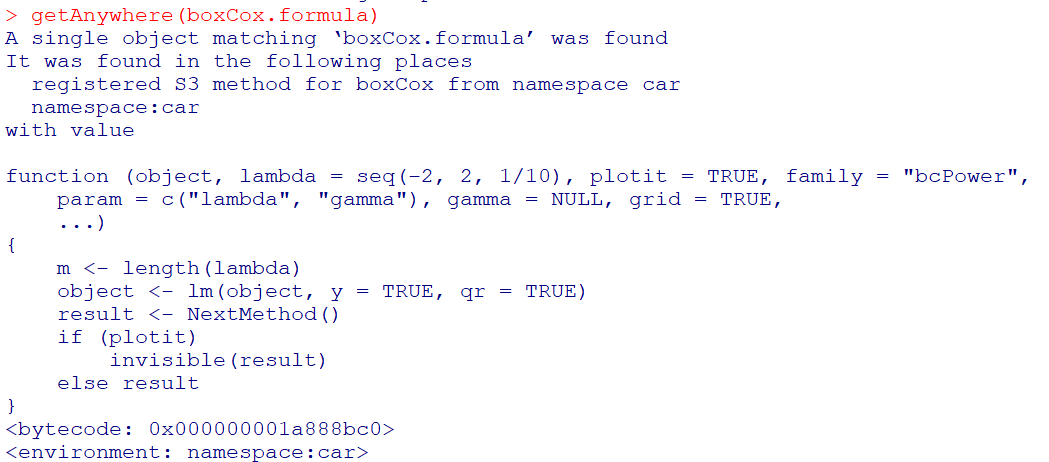Well, that is not very helpful, lets look at `getAnywhere(boxCox.default)` instead: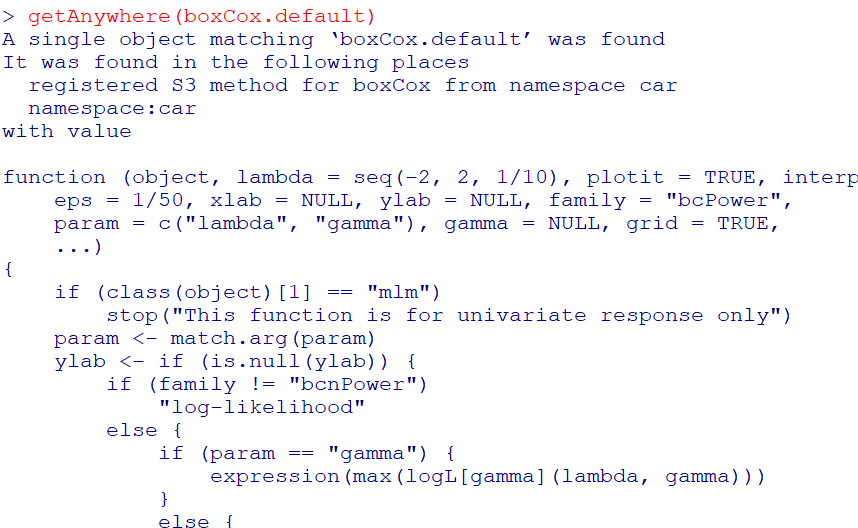Ok, that is what we are going for. If you look into the function, at the very end you will see how it draws those dashed reference lines (anything drawn with `lty = 2` in the code).

So what is happening here is that the different `boxCox` function calls are all daisy chained together, and it goes from formula -> lm object -> the original boxCox function. Now that we can see the function, we can make some small changes to have it return the locations of the vertical/horizontal reference lines that we want (or we could change it to accept a `ylim` argument directly). I name this new function `boxCox.new`.

``````# Modifying the function to return all the info you need
boxCox.new <- function(object, lambda = seq(-2, 2, 1/10), plotit = TRUE, interp = plotit,
eps = 1/50, xlab = NULL, ylab = NULL, family = "bcPower",
param = c("lambda", "gamma"), gamma = NULL, grid = TRUE,
...)
{
if (class(object) == "mlm")
stop("This function is for univariate response only")
param <- match.arg(param)
ylab <- if (is.null(ylab)) {
if (family != "bcnPower")
"log-likelihood"
else {
if (param == "gamma") {
expression(max(logL[gamma](lambda, gamma)))
}
else {
expression(max[lambda](logL(lambda, gamma)))
}
}
}
else ylab
xlab <- if (is.null(xlab)) {
if (param == "lambda")
expression(lambda)
else expression(gamma)
}
else xlab
#fam <- matchFun(family) #Needed to change this to base function
fam <- match.fun(family)
if (is.null(object\$y) || is.null(object\$qr))
stop(paste(deparse(substitute(object)), "does not have both 'qr' and 'y' components"))
y <- object\$y
n <- length(y)
xqr <- object\$qr
xl <- loglik <- if (family != "bcnPower")
as.vector(lambda)
else {
if (param == "lambda")
as.vector(lambda)
else {
if (!is.null(gamma))
as.vector(gamma)
else {
p1 <- powerTransform(object, family = "bcnPower")
gam <- p1\$gamma
se <- sd(y)
seq(max(0.01, gam - 3 * se), gam + 3 * se, length = 100)
}
}
}
m <- length(xl)
if (family != "bcnPower") {
for (i in 1L:m) {
yt <- fam(y, xl[i], j = TRUE)
loglik[i] <- -n/2 * log(sum(qr.resid(xqr, yt)^2))
}
}
else {
lambda.1d <- function(gamma) {
fn <- function(lam) bcnPowerllik(NULL, y, NULL, lambda = lam,
gamma = gamma, xqr = xqr)\$llik
f <- optimize(f = fn, interval = c(-3, 3), maximum = TRUE)
f\$objective
}
gamma.1d <- function(lambda) {
fn <- function(gam) bcnPowerllik(NULL, y, NULL, lambda = lambda,
gamma = gam, xqr = xqr)\$llik
f <- optimize(f = fn, interval = c(0.01, max(y)),
maximum = TRUE)
f\$objective
}
for (i in 1L:m) {
loglik[i] <- if (param == "lambda")
gamma.1d(loglik[i])
else lambda.1d(loglik[i])
}
}
if (interp) {
sp <- spline(xl, loglik, n = 100)
xl <- sp\$x
loglik <- sp\$y
m <- length(xl)
}
if (plotit) {
mx <- (1L:m)[loglik == max(loglik)][1L]
Lmax <- loglik[mx]
lim <- Lmax - qchisq(19/20, 1)/2
# Adding in vector to contain x functions location and top line
xF <- c()
xT <- c()
plot(xl, loglik, xlab = xlab, ylab = ylab, type = "n",
ylim = range(loglik, lim))
if (grid) {
grid(lty = 1, equilogs = FALSE)
box()
}
lines(xl, loglik)
plims <- par("usr")
abline(h = lim, lty = 2)
y0 <- plims[3L]
scal <- (1/10 * (plims[4L] - y0))/par("pin")[2L]
scx <- (1/10 * (plims[2L] - plims[1L]))/par("pin")[1L]
text(xl[1L] + scx, lim + scal, " 95%")
la <- xl[mx]
if (mx > 1 && mx < m)
segments(la, y0, la, Lmax, lty = 2)
xF <- c(xF, la)
xT <- c(xT, Lmax)
ind <- range((1L:m)[loglik > lim])
if (loglik[1L] < lim) {
i <- ind[1L]
x <- xl[i - 1] + ((lim - loglik[i - 1]) * (xl[i] -
xl[i - 1]))/(loglik[i] - loglik[i - 1])
segments(x, y0, x, lim, lty = 2)
xF <- c(xF, x)
xT <- c(xT, lim)
}
if (loglik[m] < lim) {
i <- ind[2L] + 1
x <- xl[i - 1] + ((lim - loglik[i - 1]) * (xl[i] -
xl[i - 1]))/(loglik[i] - loglik[i - 1])
segments(x, y0, x, lim, lty = 2)
xF <- c(xF, x)
xT <- c(xT, lim)
}
# See definitions of hline, vlines, vtop, ybase, just returning that info
return(list(x = xl, y = loglik, hline = lim, vlines = xF, vtop = xT, ybase = y0))
}
list(x = xl, y = loglik)
}``````

But this won’t work offhand with just calling `boxCox.new` with our same prior function calls, so we need to just entirely replace the original `boxCox.default` function for our daisy chain of function references to work. Here can use the `assignInNamespace` function to effectively overwrite the original.

``````# Need to do this to get it to work with lm objects
assignInNamespace("boxCox.default",boxCox.new,ns="car")

r1 <- with(trees, boxCox(Volume ~ log(Height) + log(Girth), data = trees))
r2 <- with(trees, boxCox(V2 ~ log(Height) + log(Girth), data = trees))``````

And now if we inspect either `r1` or `r2` you can see it returns the info we want.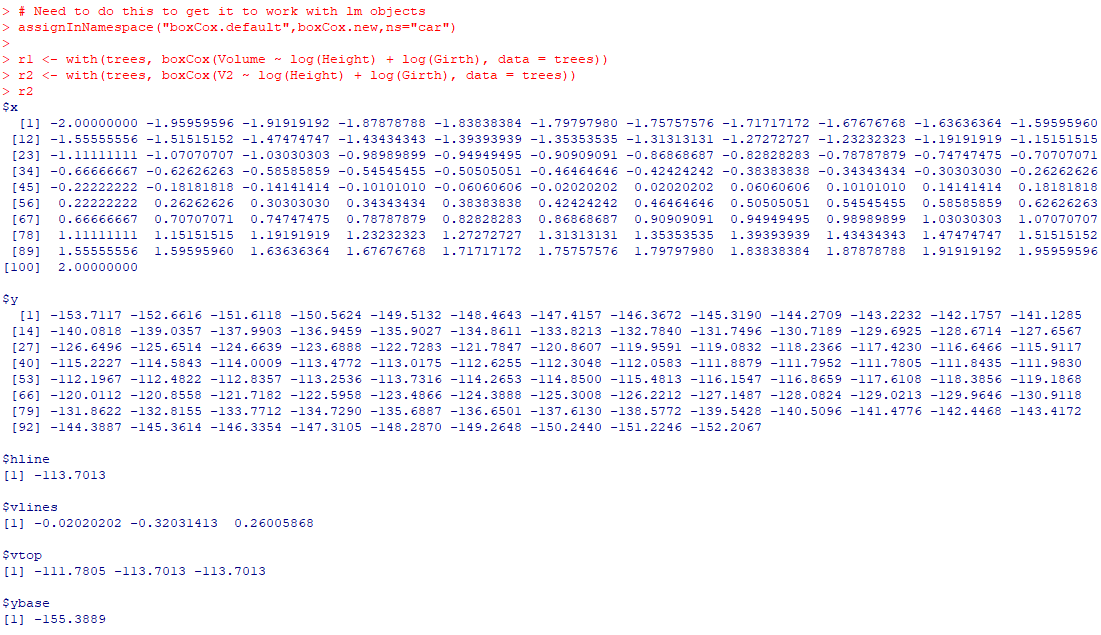And now we build own our set of plots. I don’t have the nice text annotations (or the default grid lines), but leave that to the reader to do that extra work.

``````par(mfrow=c(2,1), mai = c(1, 1, 0.2, 1))
plot(r1\$x,r1\$y,ylim=c(-160,-70), type='l', xaxp = c(-160,-70, 8),
xlab=expression(lambda),ylab='log-Likelihood')
# You need to specify the bottom of the segment to match your limit
abline(h = r1\$hline, lty = 2)
segments(r1\$vlines, -160, r1\$vlines, r1\$vtop, lty = 2)
plot(r2\$x, r2\$y,ylim=c(-160,-70), type='l', xaxp = c(-160,-70, 8),
xlab=expression(lambda),ylab='log-Likelihood')
segments(r2\$vlines, -160, r2\$vlines, r2\$vtop, lty = 2)
abline(h = r2\$hline, lty = 2)``````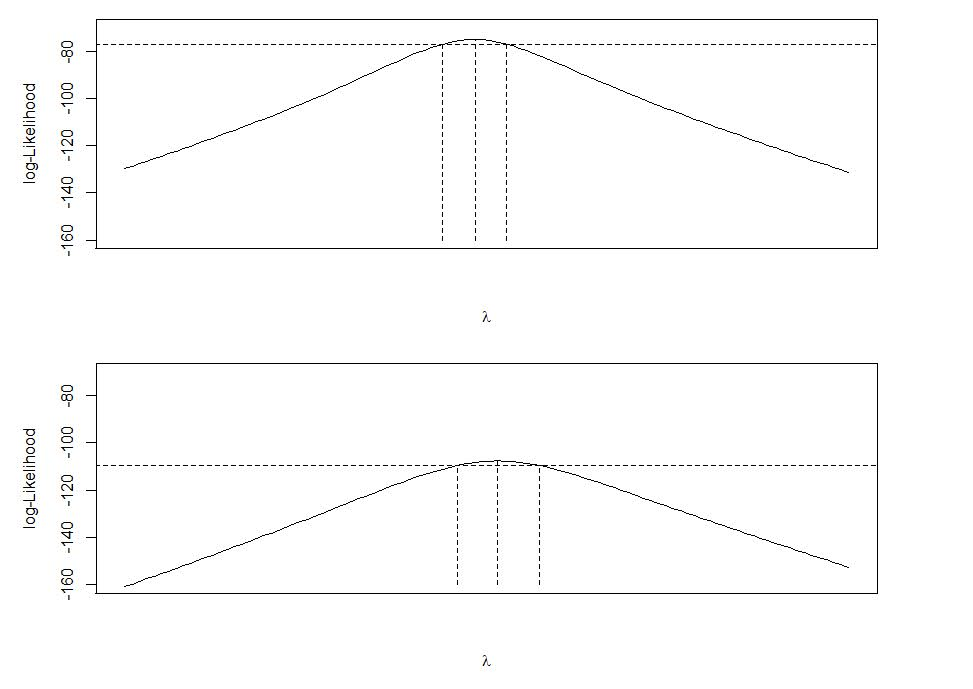I have done this previously for default plots in base R that I wanted to make myself in `ggplot`, which you could do here as well and do a facetted plot instead of the `par` deal with multiple rows (ggplot takes care of the spacing a bit nicer). But that is too much work for this quick tip to cajole those different data frames to do the facets for ggplot.